# Analumi Clock

An analogue clock without physical hands which uses UVA LED's and Photo Luminescent film.

IntermediateFull instructions provided24 hours1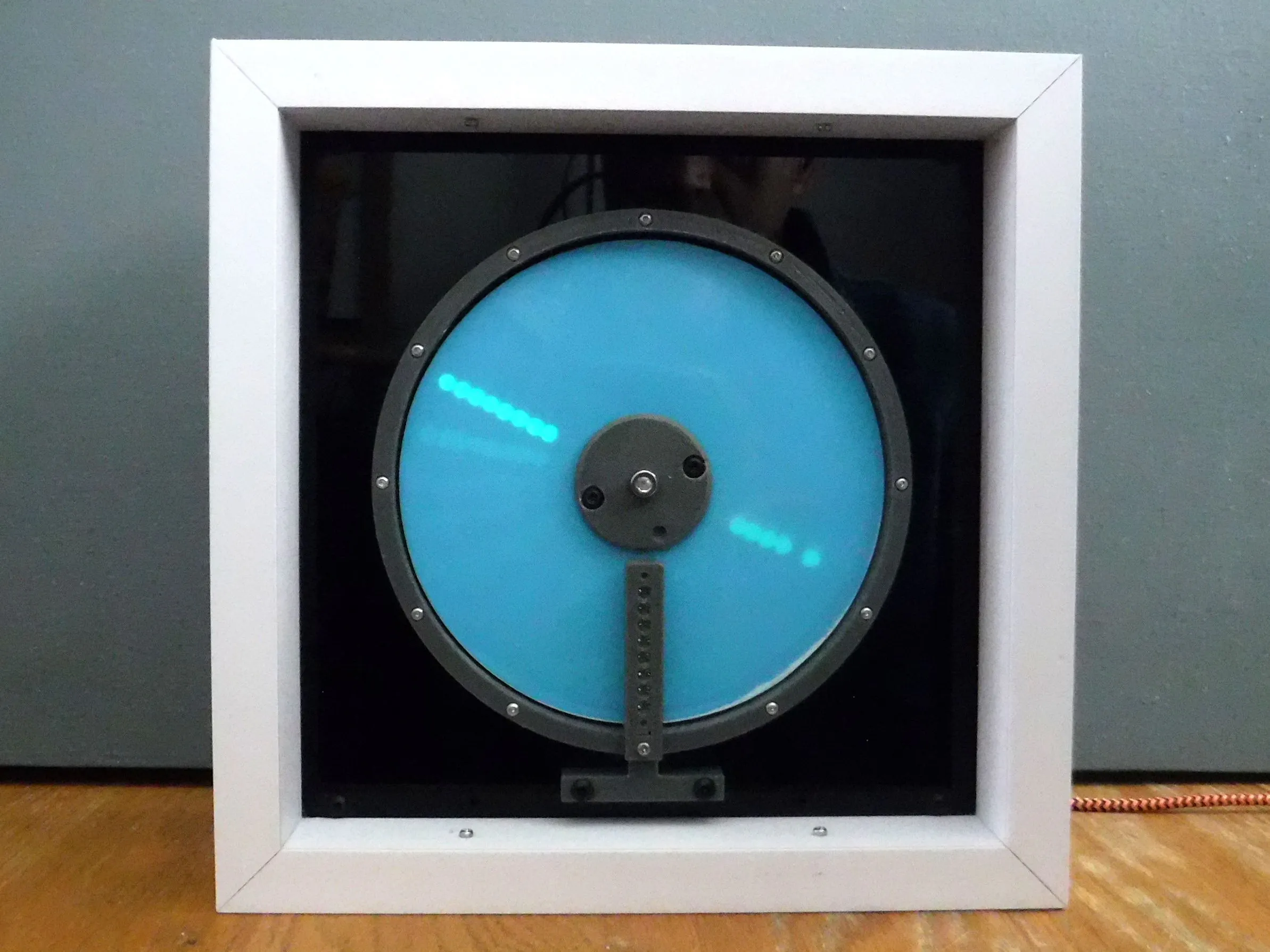## Things used in this project

### Hardware components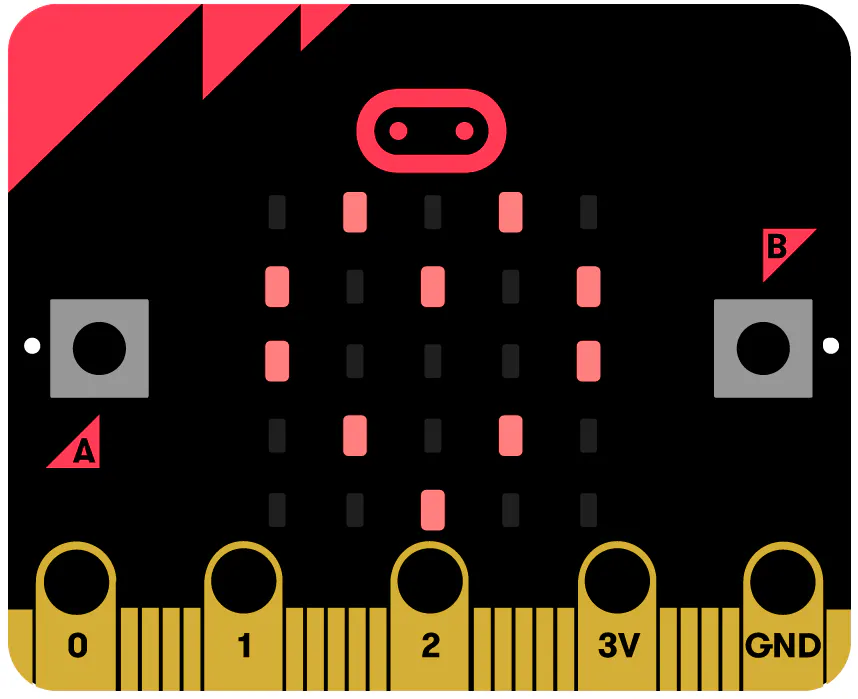BBC micro:bit board
×1
×1
 Kitronik Compact Motor Driver Board
×1
 Stepper motor and driver
×1
 Texas Instruments DRV5032FBLPG
×1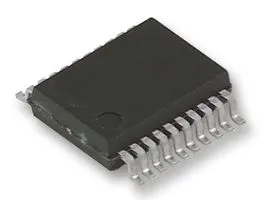Buffer / Line Driver / Line Receiver, 74HC541
×1
 UVA LED
×8
 Switch SPST
×1
 Push Button Momentary Switch
×1Resistor 10k ohm
×1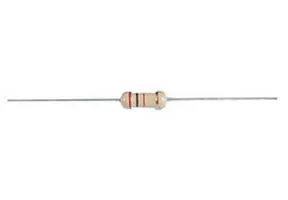Resistor 220 ohm
×8
 Luminous film
×1
 Neodymium ball magnet 3mm
×1

### Software apps and online servicesMicrosoft MakeCode

## Schematics

### Analumi Clock Schematic

The electronic elements used in the project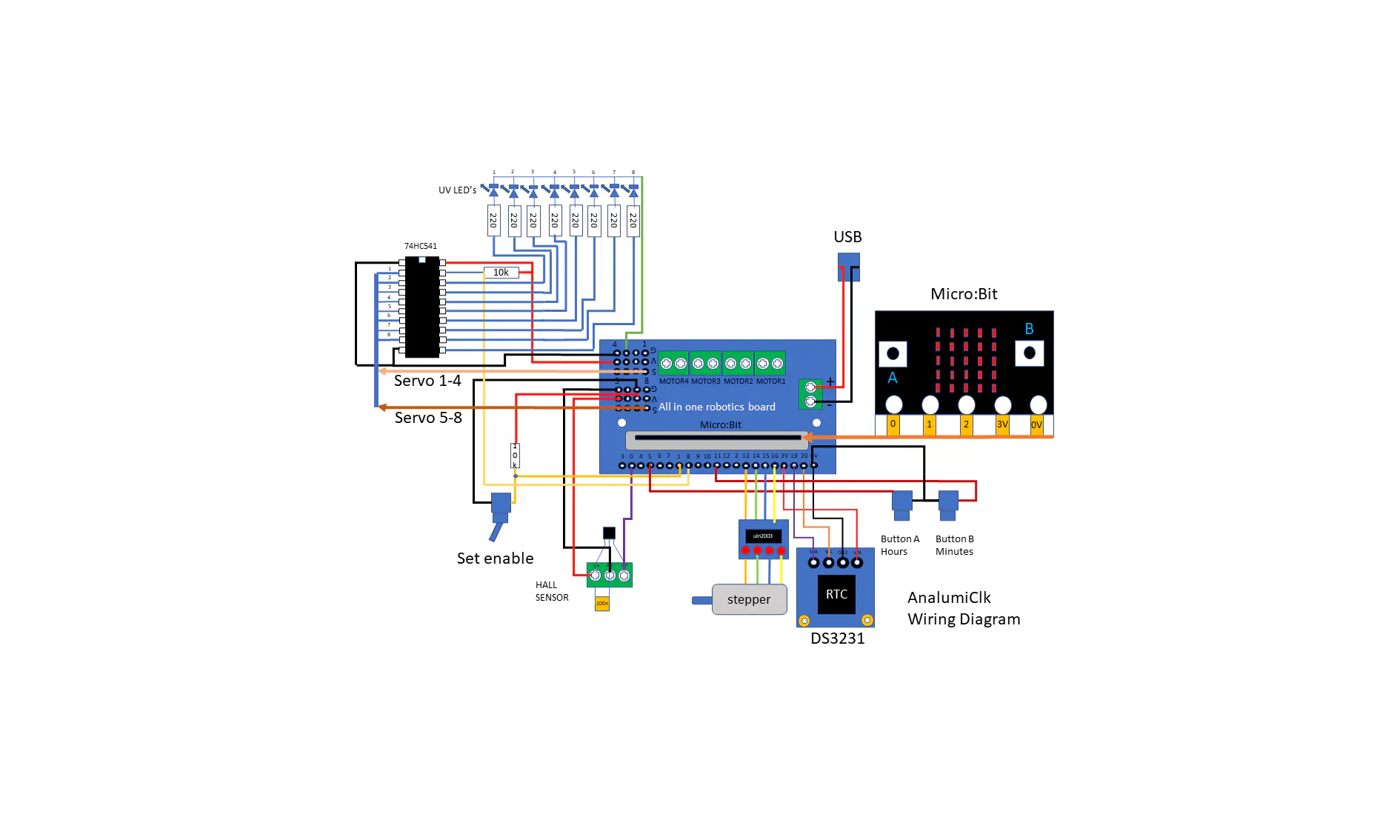## Code

### Analumi Clock Code

Python
For use with Microbit V1 (requires external sounder) or V2
```def tick():
led.plot(4, 0)
basic.pause(100)
led.unplot(4, 0)
led.toggle(4, 1)
basic.pause(100)
led.unplot(4, 1)
def anticlk():
for index in range(8):
set_bit(index)
def homing():
global home, timer, index7
home = False
timer = control.millis()
index7 = 0
while pins.digital_read_pin(DigitalPin.P0) == 1 and control.millis() - timer < 60000:
clkwise()
index7 += 1
if index7 > 512:
index7 = 0
basic.pause(100)
home = True
motor_off()
def motor_off():
pins.digital_write_pin(DigitalPin.P13, 0)
pins.digital_write_pin(DigitalPin.P14, 0)
pins.digital_write_pin(DigitalPin.P15, 0)
pins.digital_write_pin(DigitalPin.P16, 0)
def set_mode():
global set_enable
if set_enable == 0:
pass
else:
basic.show_string("+")
def auto_hrs(num: number):
home_hrs()
if home == True:
pos_hrs(num)
flash(0)
home_hrs()

def on_button_pressed_a():
global set_hr
if set_enable == 1:
set_hr += 1
if set_hr > 23:
set_hr = 0
basic.show_number(set_hr)
input.on_button_pressed(Button.A, on_button_pressed_a)

def set_bit(num2: number):
global motorbits
motorbits = beat_list[num2]
pins.digital_write_pin(DigitalPin.P13, parse_float(motorbits.substr(0, 1)))
pins.digital_write_pin(DigitalPin.P14, parse_float(motorbits.substr(1, 1)))
pins.digital_write_pin(DigitalPin.P15, parse_float(motorbits.substr(2, 1)))
pins.digital_write_pin(DigitalPin.P16, parse_float(motorbits.substr(3, 1)))
basic.pause(1.2)
def pos_min(num3: number):
global min2
basic.show_number(num3)
# steps = ((min*6)*1.42222)-256
# 512/360 = 1.422222 step/deg
min2 = int(num3 * 6 * 1.422222 - 256)
if min2 >= 0:
index2 = 0
while index2 <= abs(min2):
anticlk()
index2 += 1
else:
index3 = 0
while index3 <= abs(min2):
clkwise()
index3 += 1
def settime():
DS3231.set_time(set_hr, set_min, 0)
basic.show_number(set_hr)
basic.pause(100)
basic.show_number(set_min)
basic.pause(100)
def flash(num4: number):
global strmid
# buffer enable
pins.digital_write_pin(DigitalPin.P8, 0)
strmid = parse_float(hand_list[num4].char_at(0))
if strmid == 1:
Kitronik_Robotics_Board.servo_write(Kitronik_Robotics_Board.Servos.SERVO1, 180)
else:
Kitronik_Robotics_Board.servo_stop(Kitronik_Robotics_Board.Servos.SERVO1)
strmid = parse_float(hand_list[num4].char_at(1))
if strmid == 1:
Kitronik_Robotics_Board.servo_write(Kitronik_Robotics_Board.Servos.SERVO2, 180)
else:
Kitronik_Robotics_Board.servo_stop(Kitronik_Robotics_Board.Servos.SERVO2)
strmid = parse_float(hand_list[num4].char_at(2))
if strmid == 1:
Kitronik_Robotics_Board.servo_write(Kitronik_Robotics_Board.Servos.SERVO3, 180)
else:
Kitronik_Robotics_Board.servo_stop(Kitronik_Robotics_Board.Servos.SERVO3)
strmid = parse_float(hand_list[num4].char_at(3))
if strmid == 1:
Kitronik_Robotics_Board.servo_write(Kitronik_Robotics_Board.Servos.SERVO4, 180)
else:
Kitronik_Robotics_Board.servo_stop(Kitronik_Robotics_Board.Servos.SERVO4)
strmid = parse_float(hand_list[num4].char_at(4))
if strmid == 1:
Kitronik_Robotics_Board.servo_write(Kitronik_Robotics_Board.Servos.SERVO5, 180)
else:
Kitronik_Robotics_Board.servo_stop(Kitronik_Robotics_Board.Servos.SERVO5)
strmid = parse_float(hand_list[num4].char_at(5))
if strmid == 1:
Kitronik_Robotics_Board.servo_write(Kitronik_Robotics_Board.Servos.SERVO6, 180)
else:
Kitronik_Robotics_Board.servo_stop(Kitronik_Robotics_Board.Servos.SERVO6)
strmid = parse_float(hand_list[num4].char_at(6))
if strmid == 1:
Kitronik_Robotics_Board.servo_write(Kitronik_Robotics_Board.Servos.SERVO7, 180)
else:
Kitronik_Robotics_Board.servo_stop(Kitronik_Robotics_Board.Servos.SERVO7)
strmid = parse_float(hand_list[num4].char_at(7))
if strmid == 1:
Kitronik_Robotics_Board.servo_write(Kitronik_Robotics_Board.Servos.SERVO8, 180)
else:
Kitronik_Robotics_Board.servo_stop(Kitronik_Robotics_Board.Servos.SERVO8)
basic.pause(1000)
# buffer disable
pins.digital_write_pin(DigitalPin.P8, 1)
Kitronik_Robotics_Board.all_off()
def auto_min(num5: number):
home_min()
if home == True:
pos_min(num5)
flash(1)
home_min()
if num5 == 0:
music.play_sound_effect(music.builtin_sound_effect(soundExpression.spring),
SoundExpressionPlayMode.UNTIL_DONE)
def long_dly():
global timer
timer = control.millis()
while control.millis() - timer < 60000:
tick()
set_mode()
if set_enable == 1:
break

def on_button_pressed_ab():
if set_enable == 1:
settime()
basic.clear_screen()
input.on_button_pressed(Button.AB, on_button_pressed_ab)

def on_button_pressed_b():
global set_min
if set_enable == 1:
set_min += 1
if set_min > 59:
set_min = 0
basic.show_number(set_min)
input.on_button_pressed(Button.B, on_button_pressed_b)

def home_hrs():
basic.clear_screen()
basic.show_leds("""
. # # # .
# . # . #
# . # . #
# . . . #
. # # # .
""")
homing()
basic.clear_screen()
def pos_hrs(num6: number):
global arc, arc_plus, hr
basic.show_number(num6)
if num6 > 12:
arc = num6 - 12
else:
arc = num6
arc_plus = arc + DS3231.minutes() / 60
# steps=(((hr*30)*1.4222)-256)
# 512/360 =1.422222 steps/deg
hr = int(arc_plus * 30 * 1.422222 - 256)
if hr >= 0:
index4 = 0
while index4 <= abs(hr):
anticlk()
index4 += 1
else:
index5 = 0
while index5 <= abs(hr):
clkwise()
index5 += 1
def home_min():
basic.clear_screen()
basic.show_leds("""
. # # # .
# . . . #
# . # # #
# . . . #
. # # # .
""")
homing()
basic.clear_screen()
def clkwise():
global inc
inc = 8
for index6 in range(8):
inc += -1
set_bit(inc)
inc = 0
hr = 0
arc_plus = 0
arc = 0
strmid = 0
min2 = 0
index7 = 0
timer = 0
motorbits = ""
beat_list: List[str] = []
hand_list: List[str] = []
home = False
set_min = 0
set_hr = 0
set_enable = 0
music.set_built_in_speaker_enabled(True)
ServoBit.centre_servos()
# disable LED buffer
pins.digital_write_pin(DigitalPin.P8, 1)
motor_off()
basic.show_string("clk")
basic.pause(1000)
set_enable = 1
set_hr = 0
set_min = 0
home = False
# hour & minute hand lengths
hand_list = ["11111010", "11111111"]
# stepper motor beat list
beat_list = ["1000",
"1100",
"0100",
"0110",
"0010",
"0011",
"0001",
"1001",
"0000"]
motorbits = ""
home_hrs()

def on_forever():
set_mode()
if set_enable == 0:
if home == True:
tick()
auto_hrs(DS3231.hours())
auto_min(DS3231.minutes())
basic.clear_screen()
long_dly()
else:
basic.show_icon(IconNames.NO)
basic.forever(on_forever)
```

## Credits

### C Forde

4 projects • 1 follower